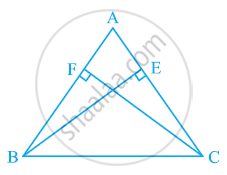# ABC is a triangle in which altitudes BE and CF to sides AC and AB are equal (see the given figure). Show that (i) ΔABE ≅ ΔACF (ii) AB = AC, i.e., ABC is an isosceles triangle. - Mathematics

ABC is a triangle in which altitudes BE and CF to sides AC and AB are equal (see the given figure). Show that

(i) ΔABE ≅ ΔACF

(ii) AB = AC, i.e., ABC is an isosceles triangle.#### Solution

(i) In ΔABE and ΔACF,

∠ABE and ∠ACF (Each 90º)

∠A = ∠A (Common angle)

BE = CF (Given)

∴ ΔABE ≅ ΔACF (By AAS congruence rule)

(ii) It has already been proved that

ΔABE ≅ ΔACF

⇒ AB = AC (By CPCT)

Concept: Properties of a Triangle
Is there an error in this question or solution?

#### APPEARS IN

NCERT Class 9 Maths
Chapter 7 Triangles
Exercise 7.2 | Q 4 | Page 124